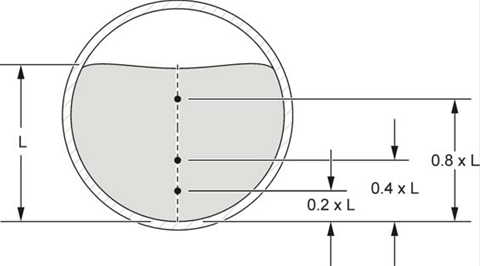# How Do You Perform the 0.2, 0.4, 0.8 Velocity Profile Method?

## Document ID

Document ID TE7892

Version 1.0

Status Published

## Published Date

Published Date 06/23/2017
Question
How Do You Perform the 0.2, 0.4, 0.8 Velocity Profile Method?
Summary
Performing the 0.2, 0.4, 0.8 Velocity Profile Method.
The 0.2, 0.4, 0.8 method is the most common method for profiling a typical flow. The velocity
is measured at three points: 0.2, 0.4, and 0.8 times the total depth of flow. The velocity from
each point is entered into the meter.

To profile the flow:
1. Measure the depth of flow.
2. Calculate the measurement positions on the center line:
• 0.2 position = 0.2 x depth of flow
• 0.4 position = 0.4 x depth of flow
• 0.8 position = 0.8 x depth of flow
3. Measure the velocities at the 0.2, .04, and 0.8 positions (Figure 13).
4. Calculate the average of the 0.2 and 0.8 velocities.
5. Calculate the average of the 0.4 velocity with the 0.2 and 0.8 average from step 4.